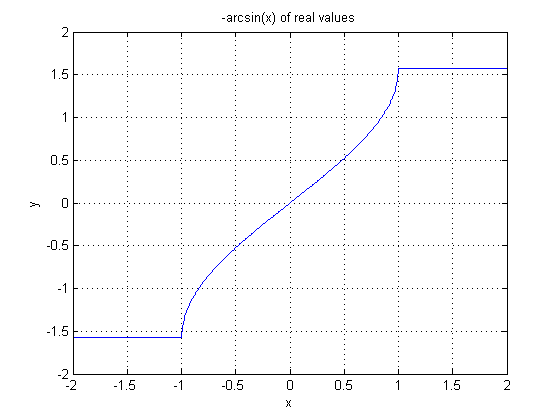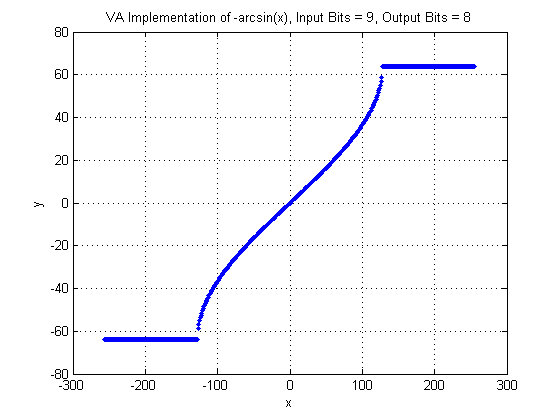## Operator ARCSIN

The operator ARCSIN is calculating the arc sine of the input.

The input range of arc sine is [-1,1]. The operator maps the input interval range [-1, 1] to where is the bit width at the input. As can be seen, not the full value range of the input is used. If an input value is outside the allowed value range, the operator will clip the value to -1 or 1. Thus, the argument x of the arc sine is determined by

The operator's output range is [-π/2, π/2]. The output value range of the operator in VisualApplets is mapped to

where

is the bit width at the output link. Thus the output value is

The following image shows the plot of the normal inverse arc sine function.In the next figure, the VisualApplets operator implementation is shown. Note the input and output bit widths.### I/O Properties

Property Value
Operator Type O

Bit Width [8, 12] [8,32]
Arithmetic signed signed
Parallelism any as I
Kernel Columns 1 as I
Kernel Rows 1 as I
Img Protocol {VALT_IMAGE2D, VALT_LINE1D, VALT_PIXEL0D} as I
Color Format VAF_GRAY as I
Color Flavor FL_NONE as I
Max. Img Width any as I
Max. Img Height any as I

None